You may also like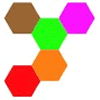Hexpentas

How many different ways can you find of fitting five hexagons together? How will you know you have found all the ways?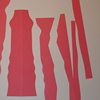Exploded Squares

This practical activity challenges you to create symmetrical designs by cutting a square into strips.

Star Find

Star Find

Here is a grid made up of $9$ squares. All of them are the same except for one. Can you find the odd one out?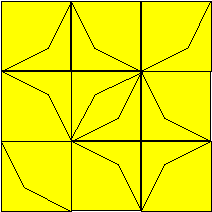The basic tile from the design above looks like this: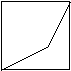In the star design, the tile has been turned around, or rotated , into different positions.
Below is a wall of $16$ blank tiles. Using the basic tile, can you make a repeating pattern to decorate our wall? Try making more designs by rotating the tile and using it in more than one position.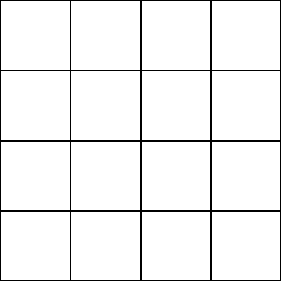Why do this problem?

This problem gives learners a chance to talk about similarities and differences, and also rotations and, in particular, quarter turns. These sheets may be useful to give to pairs or individuals:this sheet has $16$ copes of the basic tile and this sheet has a $4$ by $4$ grid which is the same size as the tiles.